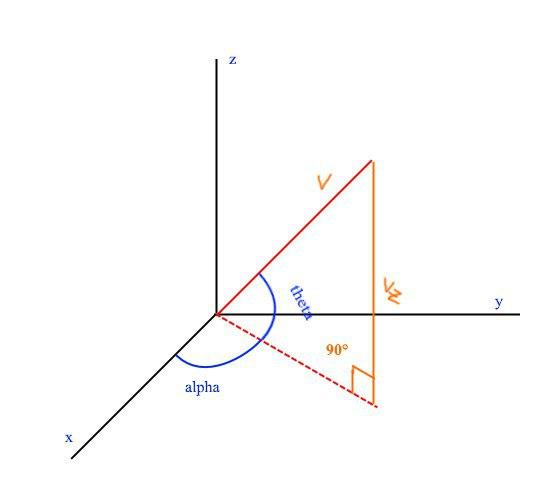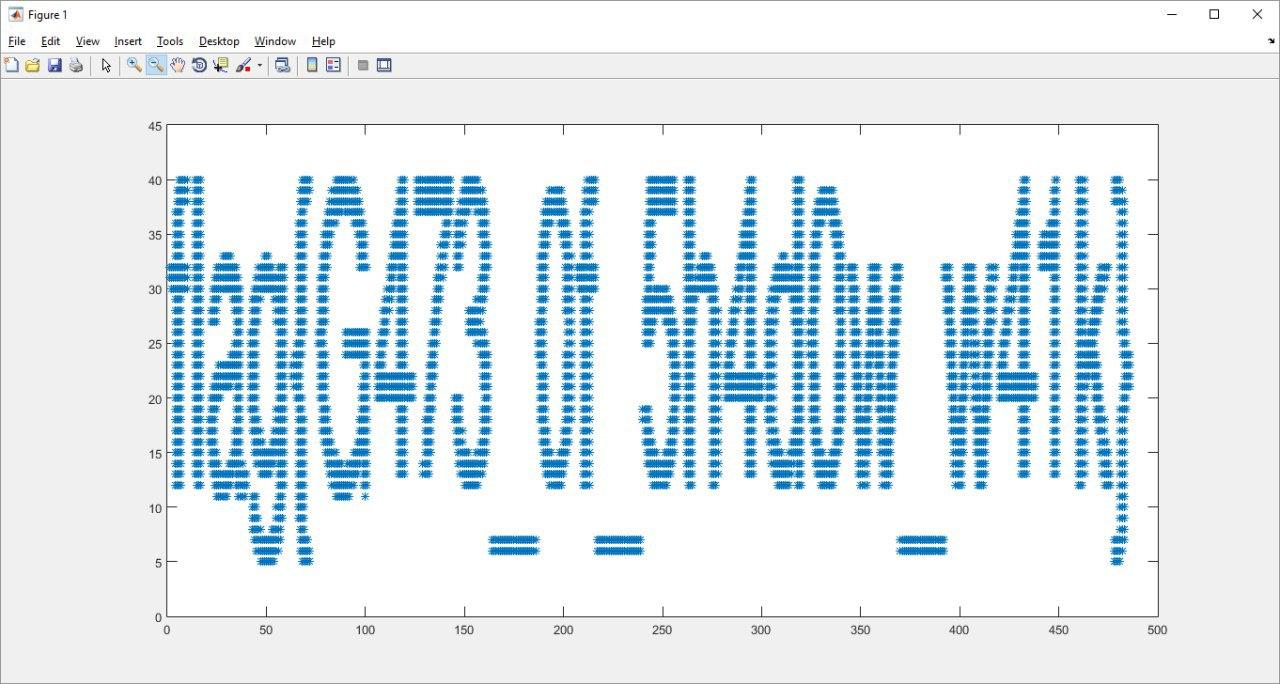### Swamp CTF - Orb2

misc
tags: ctf challenge write-up misc
by zangobot

I tried to solve different tasks, but I was not so successful. This write-up is complete from the point of view of the solution… but I didn’t managed to get the flag.

So why am I posting it?

Because I think this is worth sharing. Be patient and keep reading ;-)

The challenge is accessible via a zip folder. The password of that zip is the flag of a previous challenge. The main contents of the folder are the following files:

• a lore for the challenge;
• examples.p: a pickle file containing, for each row a tuple of five floats and a couple of int;
• page_of_numbers.p: a pickle file containing, for each row a tuple of five floats.

Mmmh. What the hell? Let’s read the lore. Be careful in reading this… because this lore matters. You’ll understand why at the bottom of this write-up.

The acolytes of several monasteries have been studying possible origins of this attack for weeks.
One of the most ancient and forbidden tomes contained in the great libraries talks about the origin of the purple dragon guard.
It appears that in ancient times, long before spellscourge, they were formidable gate keepers of Cormyr to other planes.
The most renowned branch of them fought back the great black dragon Thauglorimorgorus who was so ancient his scales turned purple.
They ended up chasing it into the Vast Swamp and founded an encampment called The Swamp Fort of Cormyr.
In this fort was a portal to the shadow plane to where they had chased Thauglor.
Those who entered never returned.  It appeared Thauglor had never been pushed back but all along was luring the militia to slaughter.
In another expedition through the portal, desperate times forced a cleric to ask a miracle of Selûne.
Our lady of silver had always been at odds with her twin, Shar, the mistress of night goddess of the plane of shadow.
The call was answered and a counterpart of this fort sprang into being with a pillar of light which extinguished the magical darkness
for miles all around.
Thauglor was repelled by this brilliant light.
This lead to many victories in this plane.  The guard resided here with the cleric at its head named The Lord of Light.
She thus forward sat on the crystal throne and lead the guardians of this shining citadel known to all as The Ever Shining Army.
This appears to be solely a legend since the only known encampment in the Vast Swamp is known as the Lost Refuge
and is rumored to house those of Church of Shar, disciples of the shadow weave.
They are nothing like those known in that legend.
It is however known that there is a fortress on the other side ruled by the Dusk Lord known as the Shadow Citadel which is situated
on the edge of the Shadow Swamp.
The mountains there are treacherous and the toxic rivers which run through the valleys sometimes lead historians to call it The Vale of Shadows.
One cleric has spent time divining a spell which determined that those pages of numbers previously found are trajectories
of the format <x position, y position, speed, angle above the horizontal, direction from the firing position>.
It appears to be that there is a spell being cast using the shadow weave and the coordinates seem to indicate
that the spells location is in the kingdom of Cormyr.


Basically, each tuple corresponds to a bullet! I have a (x,y) position, the speed of shooting, the angle above the horizontal and direction from shooting position. It’s simple 3D physics: I need to calculate the trajectory of the bullet and it’s impact point.

SPOILER: I was given a little aid by my dear brother Andrea (who is a Physicist). I learnt something new while reasoning with him. I’m not talking about calculus, formulae or physics laws, but lateral thinking in general. Having said that, let’s do some algebra.

# The physics behind this challenge

I’m going to refer to $$(x,y)$$ for the position, $$v$$ for speed, $$\theta$$ for angle above the horizontal and $$\alpha$$ for the direction. First of all, I need to understand how much time the bullet needs to fall to the ground again. I can state that:

$$v_z = v_{z0} - |g|t$$

When it will reach the ground? On the vertex of the parabule, $$v_z$$ is zero, then $$v_z$$ must be the opposite of $$v_{z0}$$ when it will touch the ground. It’s not an out-of-the-blue result, I just used the law of conservation of energy: the sum of kinetic and potential energy must be constant (there is no air friction). There will be only potential energy in the vertex of the parabule, while it is only kinetic energy at the beginning and the end of the motion.

$$v_z = -v_{z0} \\ -v_{z0} = v_{z0} - |g|t\\ t = \frac{2v_{z0}}{|g|}$$

I have now when the bullet impacts the xy plane. I need now to calculate $$v_{z0}$$:

$$v_{z0} = vsin(\theta)$$

It’s time to calculate how long the bullet will travel on x and y.I need to decompose the speed, projecting it on the $$xz$$ and $$yz$$ plane:

$$v_x = vcos(\theta)cos(\alpha)\\ v_y = vcos(\theta)sin(\alpha)\\$$

Now that I have the projections of the speed, I can calculate the impact position. I am going to use again the impact time calculated before:

$$x_f = x + v_x * t \\ y_f = y + v_y * t$$

# It’s time to Fail

Even tough the model is correct, I couldn’t match the results of examples.p file. The model calculates the trajectory of a bullet from a point $$(x,y)$$ in space to $$(x_f, y_f)$$.

## IN FACT IT WAS THE CONTRARY.

It is written clearly in the lore:

<... direction from the firing position>


So, the $$(x,y)$$ couple is an impact point, not a shooting point. Signs need to be flipped here:

$$x_f = x - v_x * t \\ y_f = y - v_y * t$$

… leading to this flag. You can find a simple script which calculates trajectory for all the points.

def predict_impact_point(row):
x,y = row,row
v = row
theta = row
alpha = row
vx = v * np.cos(theta)*np.cos(alpha)
vy = v * np.cos(theta)*np.sin(alpha)
vz = v * np.sin(theta)
g = 9.81
t = 2*vz / g
return x - vx*t, y - vy*t

matrix_data = [ ]
res = []
for d in data:
val =predict_impact_point([i for i in d])
matrix_data.append(val)
matrix_data = np.array(matrix_data)

plt.plot(matrix_data[:,0], matrix_data[:,1],'o')
plt.show()(SPOILER: there is a last trick, but I’ll let you discover by youself…)

We didn’t managed to capture the flag but we were so damn close. We had fun, that was the most important thing. Here you can download the points and script I used to solve the challenge.

See you next write-up ;)